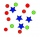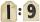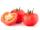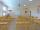Numbers

Determine the number of all positive integers less than 4183444 if each is divisible by 29, 7, 17.

What is its sum?

Result

n =  1212
S =  2536754178

Solution:Leave us a comment of this math problem and its solution (i.e. if it is still somewhat unclear...):Be the first to comment!To solve this verbal math problem are needed these knowledge from mathematics:

Need help calculate sum, simplify or multiply fractions? Try our fraction calculator.

Next similar math problems:

1. Sum of two primesChristian Goldbach, a mathematician, found out that every even number greater than 2 can be expressed as a sum of two prime numbers. Write or express 2018 as a sum of two prime numbers.
2. Divide 5Divide 288 in the following ratio 3 : 4 : 5
3. RoundingThe following numbers round to the thousandth:
4. RatioUnfold number 1963 in the ratio 9:2:2.
5. Write 3Write a real world problem involving the multiplication of a fraction and a whole number with a product that is between 8 and 10 then solve the problem
6. Nineteenth memberFind the nineteenth member of the arithmetic sequence: a1=33 d=5 find a19
7. Fractions 4How many 2/3s are in 6?
8. Watching TVOne evening 2/3 students watch TV. Of those students, 3/8 watched a reality show. Of the students that watched the show, 1/4 of them recorded it. What fraction of the students watched and recorded reality tv.
9. 6 termsFind the first six terms of the sequence. a1 = 7, an = an-1 + 6
10. Ratio 11Simplify this ratio 10 : 1/4
11. Farmers 2On Wednesday the farmers at the Grant Farm picked 2 barrels of tomatoes. Thursday, the farmers picked 1/2 as many tomatoes as on Wednesday. How many barrels of tomatoes did the farmers pick on Thursday?
12. PointsGryffindor won 437 points. How many points obtained by each of the faculties if they were split at a ratio of 5: 7: 3: 4?
13. One-third 2One-third of the people in a barangay petitioned the council to allow them to plant in vacant lots and another 1/5 of the people petitioned to have a regular garbage collection. What FRACTION of the barangay population made the petition?
14. Cupcakes 2Susi has 25 cupcakes. She gives 4/5. How much does she have left?
15. Fraction to decimalWrite the fraction 3/22 as a decimal.
16. Classroom 4In a class of 36 pupils, 2/3 are girls. How much it is in a class girls and boys?
17. Pizza 5You have 2/4 of a pizza and you want to share it equally between 2 people how much pizza does each person get?